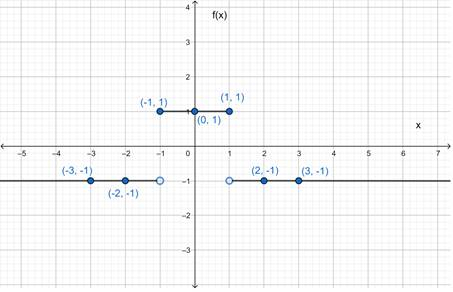# The graph of the given function.### Precalculus: Mathematics for Calcu...

6th Edition
Stewart + 5 others
Publisher: Cengage Learning
ISBN: 9780840068071### Precalculus: Mathematics for Calcu...

6th Edition
Stewart + 5 others
Publisher: Cengage Learning
ISBN: 9780840068071

#### Solutions

Chapter 2.2, Problem 39E
To determine

## To sketch : The graph of the given function.

Expert Solution

### Explanation of Solution

Given information :

f(x)={1      if  x<11        if  1x11     if x>1

Graph :

 x −3 −2 −1 0 1 2 3 f(x) −1 −1 1 1 1 −1 −1This is the graph of the given piecewise defined equation.

Interpretation : The part of the graph to the left of x=1 coincides with the graph of f(x)=1 and the part of the graph to the right of x=1 coincides with the graph of f(x)=1 . The part of the graph between x=1 and x=1 coincides with the graph of f(x)=1 . The open dots at (1,1) and (1,1) indicate that these points are excluded from the graph.

### Have a homework question?

Subscribe to bartleby learn! Ask subject matter experts 30 homework questions each month. Plus, you’ll have access to millions of step-by-step textbook answers!